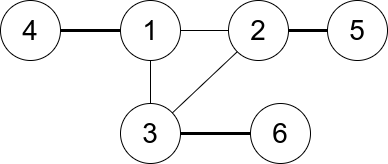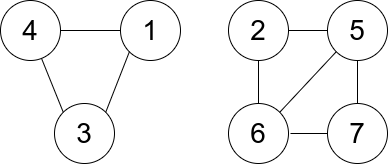# 一个图中连通三元组的最小度数

## 1761. 一个图中连通三元组的最小度数 (Hard)```输入：n = 6, edges = [[1,2],[1,3],[3,2],[4,1],[5,2],[3,6]]

``````输入：n = 7, edges = [[1,3],[4,1],[4,3],[2,5],[5,6],[6,7],[7,5],[2,6]]

1) [1,4,3]，度数为 0 。
2) [2,5,6]，度数为 2 。
3) [5,6,7]，度数为 2 。
```

• `2 <= n <= 400`
• `edges[i].length == 2`
• `1 <= edges.length <= n * (n-1) / 2`
• `1 <= ui, vi <= n`
• `ui != vi`
• 图中没有重复的边。

[]